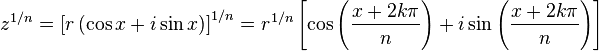# Determine the indicated roots of the complex number

• paraboloid

#### paraboloid

[2(cos(pi/3)+isin(pi/3))]1/2

I simplified it to 21/2(cos(pi/6)+isin(pi/6)), but I have no idea what else to go to.

Any tips would be very helpful,

what was the original problem? how many roots you need to find?

apart from that, you have the answer:
cos(pi/6)=sqrt(3)/2 ; sin(pi/6)=1/2

r = sqrt(2)*sqrt(3)/2 +/- i*(1/2)

that's two roots how many do you need?

Use De Moivre's formula. In general to get other roots you can add multiples of 2*pi to the angle.

what was the original problem? how many roots you need to find?

apart from that, you have the answer:
cos(pi/6)=sqrt(3)/2 ; sin(pi/6)=1/2

r = sqrt(2)*sqrt(3)/2 +/- i*(1/2)

that's two roots how many do you need?

The answer in the back is actually +/-(sqrt 3 + i) / sqrt(2). The text doesn't specify how many roots to find, but it looks like two roots.

Use De Moivre's formula. In general to get other roots you can add multiples of 2*pi to the angle.I believe I've converted it to the latter form(k = 0), but I'm still unsure what more I need to do to get the roots.

The other root is k=1. It's the negative of the first root. Since e^(i*pi)=(-1). k=2 just gives you a copy of the first root. Since e^(i*2*pi)=1.

sqrt(2)*sqrt(3)/2 +/- i*(1/2) <-- this is almost the same as:
+/-(sqrt 3 + i) / sqrt(2)

but I must say that every complex root comes in pairs! --> r=a +/- b*i
(a,b in Reals)

so if you say the answer is "+/-(sqrt 3 + i) / sqrt(2)" then it must be:
+/-(sqrt 3 + i) / sqrt(2) & +/-(sqrt 3 - i) / sqrt(2)

so you get 4 roots...

@Dick: I think he already used De Moiver's formula when he took the root and turned it into a multiplier of one-half and multiplied the term inside the cos and sin

@paraboloid if you don't know what you need to do, just read you course book or ask your Lecturer or TA.
"It's irrelevant being precise when you don't know what's going on" ! :)

sqrt(2)*sqrt(3)/2 +/- i*(1/2) <-- this is almost the same as:
+/-(sqrt 3 + i) / sqrt(2)

but I must say that every complex root comes in pairs! --> r=a +/- b*i
(a,b in Reals)

so if you say the answer is "+/-(sqrt 3 + i) / sqrt(2)" then it must be:
+/-(sqrt 3 + i) / sqrt(2) & +/-(sqrt 3 - i) / sqrt(2)

so you get 4 roots...

@Dick: I think he already used De Moiver's formula when he took the root and turned it into a multiplier of one-half and multiplied the term inside the cos and sin

@paraboloid if you don't know what you need to do, just read you course book or ask your Lecturer or TA.
"It's irrelevant being precise when you don't know what's going on" ! :)

There are only two roots. If you square the conjugates, you don't get the given number. You get it's conjugate.

There are only two roots. If you square the conjugates, you don't get the given number. You get it's conjugate.

I see why you may be right, but again the question wasn't clear and I tried to give the general direction :)

but I must say that every complex root comes in pairs! --> r=a +/- b*i
(a,b in Reals)
That's only true if the coefficients of the polynomial are real. In this problem, you're trying to find the roots of

$$z^2 - (1+i\sqrt{3}) = 0$$

which doesn't satisfy that criterion.

Thanks everyone for your input. Much appreciated.

That's only true if the coefficients of the polynomial are real. In this problem, you're trying to find the roots of

$$z^2 - (1+i\sqrt{3}) = 0$$

which doesn't satisfy that criterion.

What you are saying is right, but not in this context.
In this problem we DO NOT see the polynomial itself, but the POLAR representation of a complex number. so, as I said the number of roots depends on the original problem.
since we don't see the original polynomial a thus don't know the number of NEEDED roots !

Good luck.